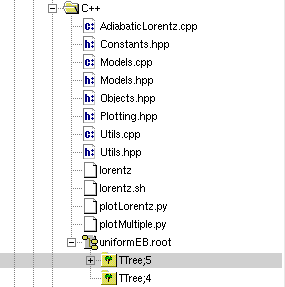# TTree creation with TBranches created in loop issue

Dear all,

I’m writing a C++ code solving the Lorentz force equation with a numerical method (from GSL) for multiple primaries, and trying to store the individual trajectories in a ROOT file. From ROOT’s documentation, I’m using TTree for storing structs of states. The following are the steps I’m performing:

• Create a ROOT file, and a TTree for the events (event = the trajectory of each particle);
• Perform the simulation for multiple particles in a loop;
• Inside the loop I create a struct containing the state of a particle at a given time (time, x, y, z, vx, vy, vz);
• The the ODE solver gives me tuples of the trajectory;
• Inside a loop for the states of each particle I create a TBranch to store the structs. It gives me the tuples x, y, z, etc as leafs.

I’m experiencing two problems:

1. Say I simulate 20 particles, with a total of 10 points in time for each (the propagation spec in the ODE solver). Then each TBranch (for each event) should contain x, y, z, etc with 10 points each. What is happening is that each leaf has then 200 points (the 10 points times the number of particles), i.e., the total number of points for the full simulation. Probably I’m looping TBranch in a wrong way(?);
2. For bigger number of primaries, TTree is divided into multiple ones, with weird number names such as TTree;4 and TTree;5, and some of them do not contain all the branches (events).

The below is my code in over the loop:

``````struct DataPoint
{
double timeStamp;
double x;
double y;
double z;
double vx;
double vy;
double vz;
};

void lorentzSimulator(int noPrimaries, struct Particle particle,
double E[], double B[], double x0[],
double v0[], double t0, double tf, double dt,
string &outputFile,
const string &ODEsolver,
bool print,
bool saveState,
const double h,
const double epsAbs,
const double epsRel)
{
string fileString = outputFile + ".root";
TFile file(fileString.c_str(), "RECREATE", "ROOT data file");
TTree tree("TTree", "data TTree");

double q = particle.charge;
double m = particle.mass;

for(int i=0; i<noPrimaries; i++){

stateVector states = lorentzSolver(q, m, E, B, x0, v0, t0, tf, dt,
ODEsolver,
h,
epsAbs,
epsRel);
dvec t  = get<0>(states);
dvec x  = get<1>(states);
dvec y  = get<2>(states);
dvec z  = get<3>(states);
dvec vx = get<4>(states);
dvec vy = get<5>(states);
dvec vz = get<6>(states);

struct DataPoint dataPoint;
string branch = "Event_" + to_string(i);
tree.Branch(branch.c_str(), &dataPoint, "t:x:y:z:vx:vy:vz");

for(uint j=0; j<x.size(); j++){
dataPoint.timeStamp = t[j];
dataPoint.x  = x[j];
dataPoint.y  = y[j];
dataPoint.z  = z[j];
dataPoint.vx = vx[j];
dataPoint.vy = vy[j];
dataPoint.vz = vz[j];
tree.Fill();
}
}
file.Write();
file.Close();
}
``````

I attach as well the prints of the branches with the total number of points, and the division of multiple trees for big number of primaries.
I’ll appreciate so much if someone can point me out where I’m doing wrong here. Thanks a lot in advance.

Br,
Leonardo._ROOT Version: 6.28
_Platform: Linux
_Compiler: gcc

I think TTree and I are confused about that loop over the primaries. Why do you create a new branch for each primary?

`TTree::Fill()` creates a new TTree entry filling the “current values” for each branch, i.e. whatever `&dataPoint` stores at the point of `TTree::Fill()`. That probably gives you an indication of a mismatch of your vs TTree’s expectationSo generally you want the same pattern for all TTree entries, and after the first `TTree::Fill()` you generally don’t want to add new branches anymore. You also don’t want multiple branches point to the same variable generally (you’d be having multiple columns storing the same values).

If none of that makes any sense maybe have a look at the basic TTree tutorials, e.g. `tutorials/tree/tree1.C`?

Dear @Axel ,

Thank you so much for the feedback. As it happens, I’m not really experienced with ROOT, and clearly I’m having a little hard time understanding TTreeSo, basically I’d like to store the trajectory of each of the primaries. By trajectory I mean each step of the integration in terms of time, position vector, and velocity vector. My initial idea is to store everything in a ROOT file, as I’m already implementing other ROOT libraries in my C++ code such as plotting and other analysis tools, which shall read the ROOT file.
The structure I had in mind was to create a TTree inside the ROOT file, with each of the events as a branch, where each branch contains the time, position, and velocity arrays. I would later on implement other TTrees to store other data from the simulations. Does that structure work at all? I attach a figure of the structure I had in mind. Otherwise, would you be kind to suggest me anything similar? I have of course taken a look at the tutorials, but none seem to cover the idea I have in mind.

And thanks again for the feedback.

Br,
Leonardo.